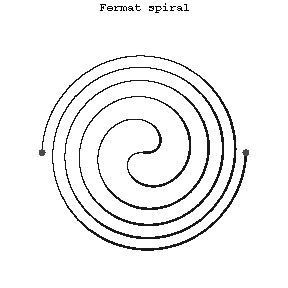# Fermat spiral

A planar transcendental curve the equation of which in polar coordinates has the form

$$\rho=a\sqrt\phi.$$

To each value of $\phi$ correspond two values of $\rho$ — a positive and a negative one. The Fermat spiral is centrally symmetric relative to the pole, which is a point of inflection. It belongs to the class of so-called algebraic spirals.

They were first studied by P. Fermat (1636).Figure: f038420a

How to Cite This Entry:
Fermat spiral. Encyclopedia of Mathematics. URL: http://encyclopediaofmath.org/index.php?title=Fermat_spiral&oldid=32537
This article was adapted from an original article by D.D. Sokolov (originator), which appeared in Encyclopedia of Mathematics - ISBN 1402006098. See original article